# SIN Excel FunctionArticle byExcelMojo Team## What Is SIN Excel Function?

The SIN Excel Function calculates the SINE value of the given angle. To calculate the SIN function, the input values must be compatible with the formula, else we can use the Radians function or convert to the angle to degrees using the expression [PI()/180].

The SIN function is an inbuilt “Math and Trig” function, so we can insert the formula from the “Function Library” or enter it directly in the worksheet.

For example, the image below depicts the Value in column A. We will apply the SIN function to calculate the SIN values.

Select cell B2, enter the SIN Excel formula =SIN(A2 * PI() / 180), and press “Enter”.

The result is ‘0.866’, as shown above. Column C is for our reference.

###### Key Takeaways
• The SIN Excel function is a trigonometric function that returns the SINE of an angle.
• The SIN function accepts the SIN angle values in degrees. If the values are not in the required way, we can convert the values to degrees, i.e., for ex. 80 to 80o, using the expression [PI()/180], or use the RADIANS function.
• To calculate the SINE value, we use the SIN() function along with other functions such as PI(), RADIANS(), DEGREES(), etc.
• The PI() function helps us derive the accurate results while working with π.

### SIN() Excel Formula

The syntax of the SIN Excel formula is,

The argument of the SIN Excel formula is,

• number: It is a mandatory argument. The angle in radians for which we want the SINE.

### How To Use SIN Excel Function?

We can use the SIN function using 2 ways, namely:

1. Access from the Excel ribbon.
2. Enter in the worksheet manually.

#### Method #1 – Access from the Excel ribbon

Choose an empty cell for the result → select the “Formulas” tab → go to the “Function Library” group → click the “Math & Trig” option drop-down → select the “SIN” function, as shown below.

The “Function Arguments” window appears. Enter the arguments in the “Number” field, and click “OK”, as shown below.

#### Method #2 – Enter in the worksheet manually

1. Select an empty cell for the output.
2. Type =SIN( in the selected cell. [Alternatively, type =S or =SI and double-click the SIN function from the list of suggestions shown by Excel.
3. Enter the argument as cell value or cell reference in excel and close the brackets.
4. Press the “Enter” key.

The succeeding example depicts the values, and we will calculate the SIN value using the SIN Excel function combined with the RADIANS function.

In the table, the data is,

• Column A contains the Value.
• Column B contains the Result.

The steps to calculate the value using the SIN excel formula are,

1. Select cell B2, and enter the formula =SIN(RADIANS, i.e., the value of the Radian function.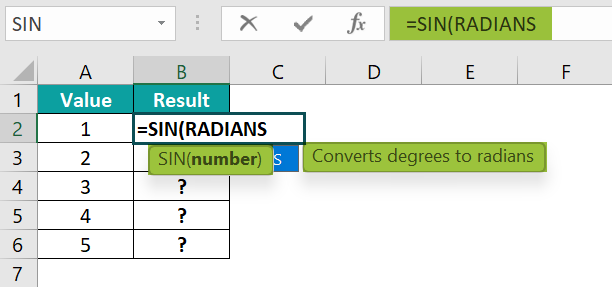2. Enter the value of the ‘angle’ as A2, and close the brackets. The complete formula is =SIN(RADIANS(A2))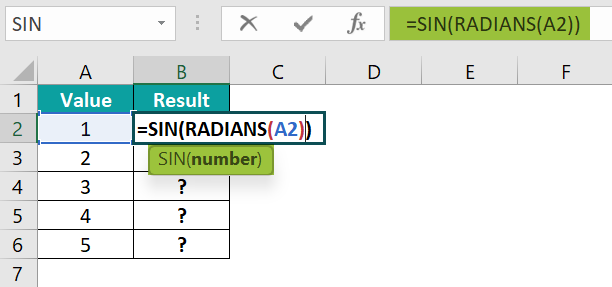3. Press the “Enter” key. The result is “0.0175”, as shown below.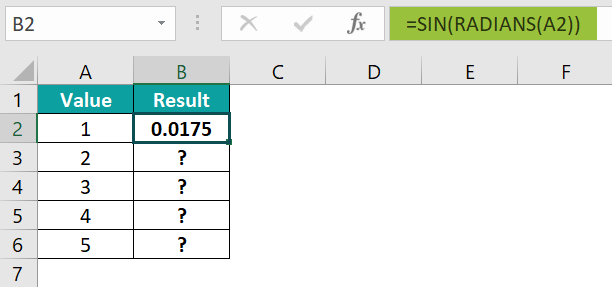4. Drag the formula from cell B2 to B6 using the fill handle. The output is shown below.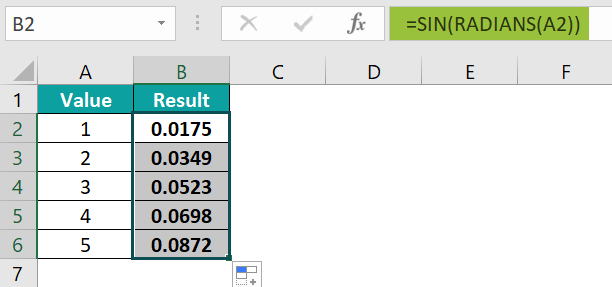### Examples

We will consider some advanced scenarios using the SIN Excel function examples.

#### Example #1

The succeeding example depicts the values, and we will calculate the SIN value using the SIN and the RADIANS functions.

In the table, the data is,

• Column A contains the Value.
• Column B contains the Result.

The steps to calculate the value using the SIN formula are,

• Step 1: Select cell B2, enter the formula =SIN(RADIANS(A2)), and press the “Enter” key. The result is “-0.819”, as shown below.
• Step 2: Drag the formula from cell B2 to B6 using the fill handle. The output is shown below.

#### Example #2

The succeeding example depicts the values, and we will calculate the SIN value using the SIN and the DEGREES function.

In the table, the data is,

• Column A contains the Value.
• Column B contains the Result.

The steps to calculate the value using the SIN formula are,

• Step 1: Select cell B2, enter the formula =SIN(DEGREES(A2)), and press the “Enter” key. The result is “0.9276”, as shown below.
• Step 2: Drag the formula from cell B2 to B6 using the fill handle in excel. The output is shown below.

#### Example #3

The succeeding example depicts the values, and we will calculate the SIN value using the SIN, PI excel function, and RADIANS functions.

In the table, the data is,

• Column A contains the Value.
• Column B contains the Result.
• Column C contains the Formula.

The procedure to calculate the value using the SIN formula is,

Enter the formulas as follows:

• =SIN(PI()) in cell B2,
• =SIN(PI() /2) in cell B3,
• =SIN(A2 * PI() / 180) in cell B4, and
• After entering each formula, press the “Enter” key.

The results are shown above from cells B2 to B5. Column C is for our reference.

#### Example #4

The succeeding example depicts the values, and we will calculate the SIN value using the SIN and the DEGREES function.

In the table, the data is,

• Column A contains the Value.
• Column B contains the Result.

The steps to calculate the value using the SIN formula are,

• Step 1: Select cell B2, enter the formula =SIN(DEGREES(A2)), and press the “Enter” key. The result is “-0.014”, as shown below.
• Step 2: Drag the formula from cell B2 to B6 using the fill handle. The output is shown below.

### Important Things To Note

• We get the “#VALUE!” error when the selected cell value or cell reference is non-numeric.
• If the cell value is blank, then, by default, the formula will return the output as 0.
• We get the “#NAME?” error when the function name is incorrect.

1. What does the SIN function do in Excel?

The SIN function is a trigonometric function to calculate the SINE value that uses radians & degrees to calculate.

The syntax of the SIN function is =SIN(number)

2. How does the SIN function work?

The Excel SIN function works in Excel as follows:
1) Select an empty cell for the output.
2) Type =SIN( in the selected cell. [Alternatively, type =S or =SI and double-click the SIN function from the list of suggestions shown by Excel.
3) Enter the argument as cell value or cell reference and close the brackets.
4) Press the “Enter” key.

For example, the image depicts the values, and we will calculate the SIN value of the given values using the SIN Function.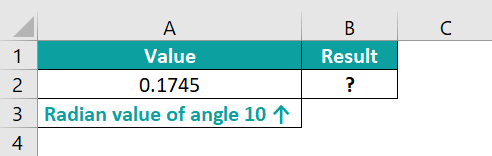The procedure to calculate the value using the SIN formula is,

Select cell B2, enter the formula=SIN(A2), and press “Enter”.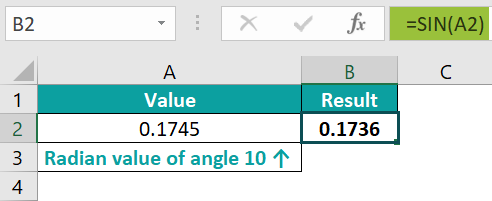The result is “0.1736”, as shown above.

3. Where is the SIN function in Excel?

The SIN function in Excel is in the Formulas tab, as shown below.

Choose an empty cell for the result → select the “Formulas” tab → go to the “Function Library” group → click the “Math & Trig” option drop-down → select the “SIN” function, as shown below.